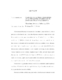## Variable Selection Properties of L1 Penalized Regression in Generalized Linear Models##### Files
umi-umd-5921.pdf(484.68 KB)
A hierarchical Bayesian formulation in Generalized Linear Models (GLMs) is proposed in this dissertation. Under this Bayesian framework, empirical and fully Bayes variable selection procedures related to Least Absolute Selection and Shrinkage Operator (LASSO) are developed. By specifying a double exponential prior for the covariate coefficients and prior probabilities for each candidate model, the posterior distribution of candidate model given data is closely related to LASSO, which shrinks some coefficient estimates to zero, thereby performing variable selection. Various variable selection criteria, empirical Bayes (CML) and fully Bayes under the conjugate prior (FBC\_Conj), with flat prior (FBC\_Flat) a special case, are given explicitly for linear, logistic and Poisson models. Our priors are data dependent, so we are performing a version of objective Bayes analysis. Consistency of $L_p$ penalized estimators in GLMs is established under regularity conditions. We also derive the limiting distribution of $\sqrt{n}$ times the estimation error for $L_p$ penalized estimators in GLMs. Simulation studies and data analysis results of the Bayesian criteria mentioned above are carried out. They are also compared to the popular information criteria, Cp, AIC and BIC. The simulations yield the following findings. The Bayesian criteria behave very differently in linear, Poisson and logistic models. For logistic models, the performance of CML is very impressive, but it seldom does any variable selection in Poisson cases. The CML performance in the linear case is somewhere in between. In the presence of a predictor coefficient nearly zero and some significant predictors, CML picks out the significant predictors most of the time in the logistic case and fairly often in the linear case, while FBC\_Conj tends to select the significant predictors equally well in all linear, Poisson and logistic models. The behavior of fully Bayes criteria depends strongly on their chosen priors for the Poisson and logistic cases, but not in the linear case. From the simulation studies, the Bayesian criteria are generally more likely than Cp and AIC to choose correct predictors. Keywords: Variable Selection; Generalized Linear Models; Hierarchical Bayes Formulation; Least Absolute Shrinkage and Selection Operator (LASSO); Information criteria; $L_p$ penalty; Asymptotic theory